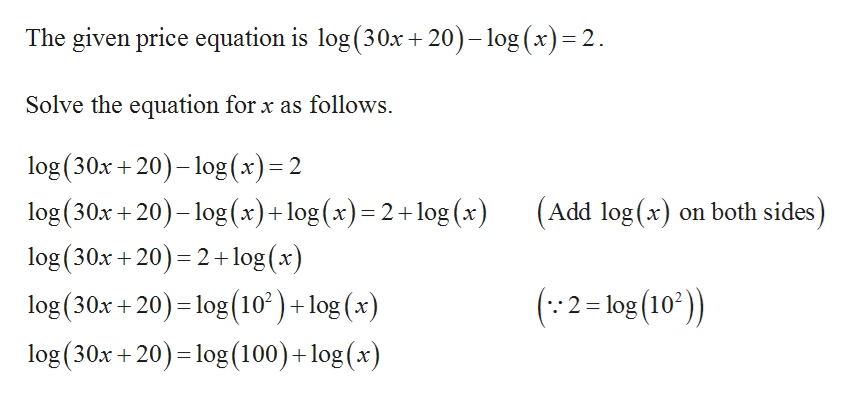# Solve for x log (30x+20)- log(x)=2

Question
6 views

Solve for x log (30x+20)- log(x)=2

check_circle

Step 1help_outlineImage TranscriptioncloseThe given price equation is log (30x+20)-log (x) 2. Solve the equation for x as follows log (30x +20)-log (x)= 2 log (30x 20)-log(x)+log (x)= 2+ log (x) log(30x +20) 2+1log (x) log (30x +20) log (10)+ log (x) log (30x +20) log (100)+ log (x) (Add log (x) on both sides) (2=log(10)) fullscreen

### Want to see the full answer?

See Solution

#### Want to see this answer and more?

Solutions are written by subject experts who are available 24/7. Questions are typically answered within 1 hour.*

See Solution
*Response times may vary by subject and question.
Tagged in

### Other